## Solving SBT Lesson 4: Multiplication and division of one-variable polynomials (C7 SBT Math 7 Horizons) – Math Book

Solving SBT Lesson 4: Multiplication and division of one-variable polynomials (C7 SBT Math 7 Horizons)
===========

### Solve problem 1 page 32 SBT Math 7 Creative horizon episode 2 – CTST

Multiply $$\left( {7x – 2} \right)\left( { – 2x + 5} \right)$$.

Detailed instructions for solving Lesson 1

Solution method

Understand the one-variable polynomial rule: To multiply a polynomial by a polynomial, we multiply each term of this polynomial by each term of the other polynomial and then add the products together.

Detailed explanation

$$\left( {7x – 2} \right)\left( { – 2x + 5} \right) = 7x\left( { – 2x} \right) + 7x.5 + \left( { – 2} \ right)\left( { – 2x} \right) + \left( { – 2} \right).5 = – 14{x^2} + 39x – 10$$

–>

— *****

### Solution 2 page 32 SBT Math 7 Creative horizon episode 2 – CTST

Multiply $$\left( {3x – 4} \right)\left( { – 2{x^2} + 7x + 4} \right)$$.

Detailed instructions for solving Lesson 2

Solution method

Understand the rule of one-variable polynomials: To multiply a polynomial by a polynomial, we multiply each term of one polynomial by each term of the other polynomial and then add the products together.

Detailed explanation

$$\begin{array}{l}\left( {3x – 4} \right)\left( { – 2{x^2} + 7x + 4} \right)\\ = 3x\left( { – 2 {x^2}} \right) + 3x.7x + 3x.4 + \left( { – 4} \right).\left( { – 2{x^2}} \right) + \left( { – 4} \right).7x + \left( { – 4} \right).4\\ = – 6{x^3} + 21{x^2} + 12x + 8{x^2} – 28x – 16 \\ = – 6{x^3} + 29{x^2} – 16x – 16\end{array}$$

–>

— *****

### Solution 3 page 32 SBT Math 7 Creative horizon episode 2 – CTST

Multiply $$\left( {4{x^2} – 2x + 1} \right)\left( { – 2{x^2} + 5x + 3} \right)$$.

Detailed instructions for solving Lesson 3

Solution method

Understand the rule of one-variable polynomials: To multiply a polynomial by a polynomial, we multiply each term of one polynomial by each term of the other polynomial and then add the products together.

Detailed explanation

$$\begin{array}{l}\left( {4{x^2} – 2x + 1} \right)\left( { – 2{x^2} + 5x + 3} \right)\\ = 4{x^2}\left( { – 2{x^2}} \right) + 4{x^2}.5x + 4{x^2}.3 – 2x.\left( { – 2{x ^2}} \right) – 2x.5x – 2x.3 + \left( { – 2{x^2}} \right) + 5x + 3\\ = – 8{x^4} + 20{x^ 3} + 12{x^2} + 4{x^3} – 10{x^2} – 6x – 2{x^2} + 5x + 3\\ = – 8{x^4} + 24{x ^3} – x + 3\end{array}$$

–>

— *****

### Solution 4 page 33 SBT Math 7 Creative horizon episode 2 – CTST

Make a polynomial expression in the variable $$x$$ representing the area of ​​the shaded part in Figure 1.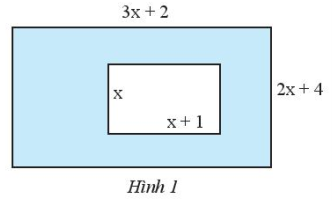Detailed instructions for solving Lesson 4

Solution method

Use the learned area formulas to write the expression.

Area of ​​a rectangle is the product of length and width

Detailed explanation

The area of ​​the large rectangle is $$\left( {3x + 2} \right)\left( {2x + 4} \right) = 2x.3x + 2x.2 + 4.3x + 4.2 = 6{x^2 } + 16x + 8$$.

The area of ​​the small rectangle is $$x\left( {x + 1} \right) = {x^2} + x$$.

The required area is $$6{x^2} + 16x + 8 – \left( {{x^2} + x} \right) = 5{x^2} + 15x + 8$$.

–>

— *****

### Solve problem 5 page 33 SBT Math 7 Creative horizon episode 2 – CTST

Do the division $$\left( {9{x^5} – 15{x^4} + 27{x^3} – 12{x^2}} \right):3{x^2}$$ .

Detailed instructions for solving Lesson 5

Solution method

Polynomial division by monomial: Divide each term of the polynomial by the monomial and add the quotients together.

Detailed explanation

$$\begin{array}{l}\left( {9{x^5} – 15{x^4} + 27{x^3} – 12{x^2}} \right):3{x^ 2}\\ = 9{x^5}:3{x^2} + \left( { – 15{x^4}} \right):3{x^2} + 27{x^3}:3 {x^2} + \left( { – 12{x^2}} \right):3{x^2}\\ = 3{x^3} – 5{x^2} + 9x – 4\end {array}$$

–>

— *****

### Solution 6 page 33 SBT Math 7 Creative horizon episode 2 – CTST

Do the division $$\left( {2{x^2} – 5x + 3} \right):\left( {2x – 3} \right)$$.

Detailed instructions for solving Lesson 6

Solution method

Divide polynomials divide polynomials by setting the division calculation.

Detailed explanation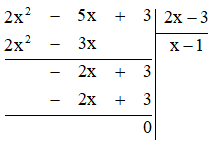So $$\left( {2{x^2} – 5x + 3} \right):\left( {2x – 3} \right) = x – 1$$

–>

— *****

### Solution 7 page 33 SBT Math 7 Creative horizon episode 2 – CTST

Do the division $$\left( {4{x^2} – 5} \right):\left( {x – 2} \right)$$.

Detailed instructions for solving Lesson 7

Solution method

Divide polynomials divide polynomials by setting the division calculation.

Detailed explanation

We perform polynomial division: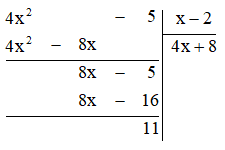So $$\frac{{4{x^2} – 5}}{{x – 2}} = 4x + 8 + \frac{{11}}{{x – 2}}$$.

–>

— *****

### Solve problem 8 page 33 SBT Math 7 Creative horizon episode 2 – CTST

Do the division $$\left( {4{x^3} – 7x + 2} \right):\left( {2{x^2} – 3} \right)$$.

Detailed instructions for solving lesson 8

Solution method

Divide polynomials divide polynomials by setting the division calculation.

Detailed explanation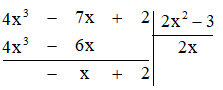So $$\frac{{4{x^3} – 7x + 2}}{{2{x^2} – 3}} = 2x + \frac{{ – x + 2}}{{2{x^) 2} – 3}}$$

–>

— *****

### Solve lesson 9 page 33 SBT Math 7 Creative horizon episode 2 – CTST

Calculate the length of a rectangle whose area is $$4{y^2} + 4y – 3$$ (cm2) and the width is equal to $$\left( {2y – 1} \right)$$(cm).

Detailed instructions for solving Lesson 9

Solution method

The length of the rectangle is the quotient of the area divided by the width.

Detailed explanation

We have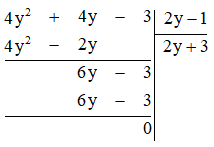So the length of the given rectangle is $$2y + 3$$ cm.

–>

— *****

### Solution 10 page 33 SBT Math 7 Creative horizon episode 2 – CTST

Given a rectangular box with volume $$V = 3{x^3} + 8{x^2} – 45x – 50$$ (cm3), length is equal to $$\left( {x + 5} \right)$$ cm and height $$\left( {x + 1} \right)$$ cm. Calculate the width of the rectangular box.

Detailed instructions for solving Lesson 10

Solution method

The volume of a rectangular box is the product of the three dimensions.

Width equals volume divided by product of length and height

Detailed explanation

We have the width calculated by the calculation:

$$\left( {3{x^3} + 8{x^2} – 45x – 50} \right):\left[ {\left( {x + 5} \right)\left( {x + 1} \right)} \right] = \left( {3{x^3} + 8{x^2} – 45x – 50} \right):\left( {{x^2} + 6x + 5} \right)$$

We have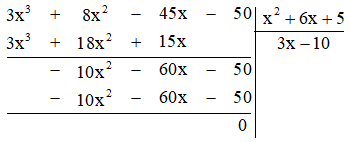So the width of the rectangle is $$3x – 10$$ cm.

–>

— *****